8DC_generator_and_an_electric_engine_Worksheet1
Оценка 4.8

# 8DC_generator_and_an_electric_engine_Worksheet1

Оценка 4.8
docx
07.05.2020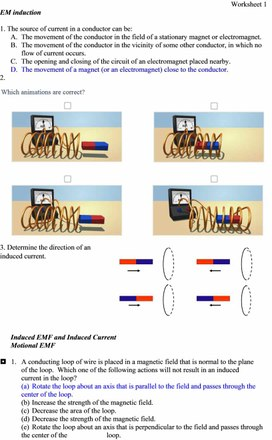8DC_generator_and_an_electric_engine_Worksheet1.docx

Worksheet 1

EM induction

1. The source of current in a conductor can be:

A.    The movement of the conductor in the field of a stationary magnet or electromagnet.

B.     The movement of the conductor in the vicinity of some other conductor, in which no flow of current occurs.

C.     The opening and closing of the circuit of an electromagnet placed nearby.

D.    The movement of a magnet (or an electromagnet) close to the conductor.

2.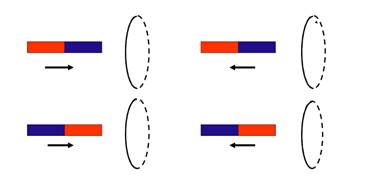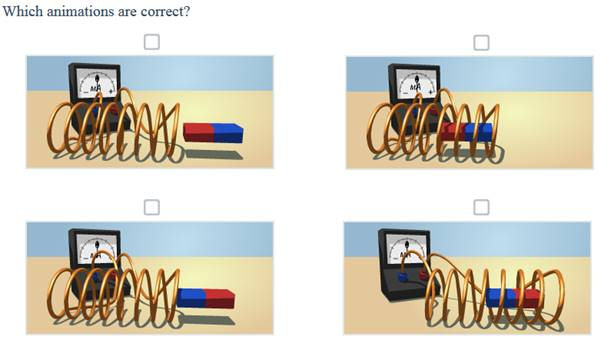3. Determine the direction of an induced current.

Induced EMF and Induced Current

Motional EMF

¦   1.   A conducting loop of wire is placed in a magnetic field that is normal to the plane of the loop.  Which one of the following actions will not result in an induced current in the loop?

(a)  Rotate the loop about an axis that is parallel to the field and passes through the center of the loop.

(b)  Increase the strength of the magnetic field.

(c)  Decrease the area of the loop.

(d) Decrease the strength of the magnetic field.

(e)  Rotate the loop about an axis that is perpendicular to the field and passes through the center of the       loop.

¢   2.   Which of the following units are equivalent to those of motional emf?

(a)  T × m/s                               (c)  J/s                                      (e)  T × m

(b)  V × m2/s                             (d) kg × m2/(C × s2)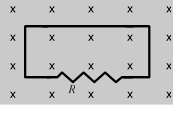¦   3.   The figure shows a uniform magnetic field that is normal to the plane of a conducting loop, which has a resistance R.  Which one of the following changes will cause an induced current to flow through the resistor?

(a)  decreasing the area of the loop

(b)  decreasing the magnitude of the magnetic field

(c)  increasing the magnitude of the magnetic field

(d) rotating the loop through 90° about an axis in the plane of the paper

(e)  all of the above

 ¦   4.   A conducting bar moves to the left at a constant speed v on two conducting rails joined at the left as shown.  As a result of the bar moving through a constant magnetic field, a current I is induced in the indicated direction.  Which one of the following directions is that of the magnetic field?       (a)  toward the right     (d) into the page       (b)  toward the left       (e)  out of the page       (c)  parallel to the long axis of the bar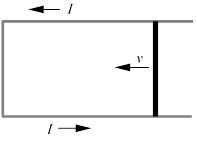¢   5.   A 2.0-kg rod that has a length of 1.0 m and a resistance of 4.0 slides with constant speed down a pair of frictionless vertical conducting rails that are joined at the bottom.  Other than the rod, the rest of the circuit is resistanceless.  A uniform magnetic field of magnitude 3.0 T is perpendicular to the plane formed by the rod and the rails as shown.  Determine the speed of the rod.       (a)  0.38 m/s                             (d) 5.6 m/s       (b)  0.90 m/s                             (e)  8.7 m/s       (c)  2.6 m/s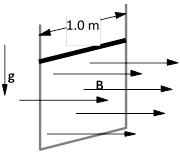¦   6.   A 0.45-m metal rod moves 0.11 m in a direction that is perpendicular to a 0.80-T magnetic field in an elapsed time of 0.036 s.  Assuming that the acceleration of the rod is zero m/s2, determine the emf that exists between the ends of the rod.

(a)  1.1 V                                 (d) 9.1 ´ 10–5 V

(b)  0.27 V                               (e)  This cannot be determined without knowing the

(c)  0.076 V                                   orientation of the rod relative to the magnetic field.

Key

3. The direction of an induced current determines using the Gimlet rule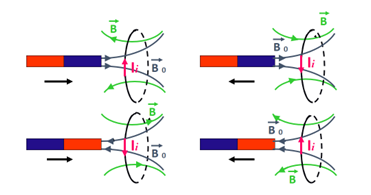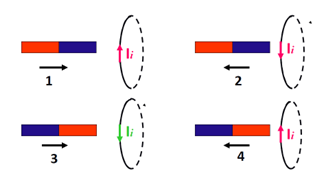## Which of the following units are equivalent to those of motional emf? (a)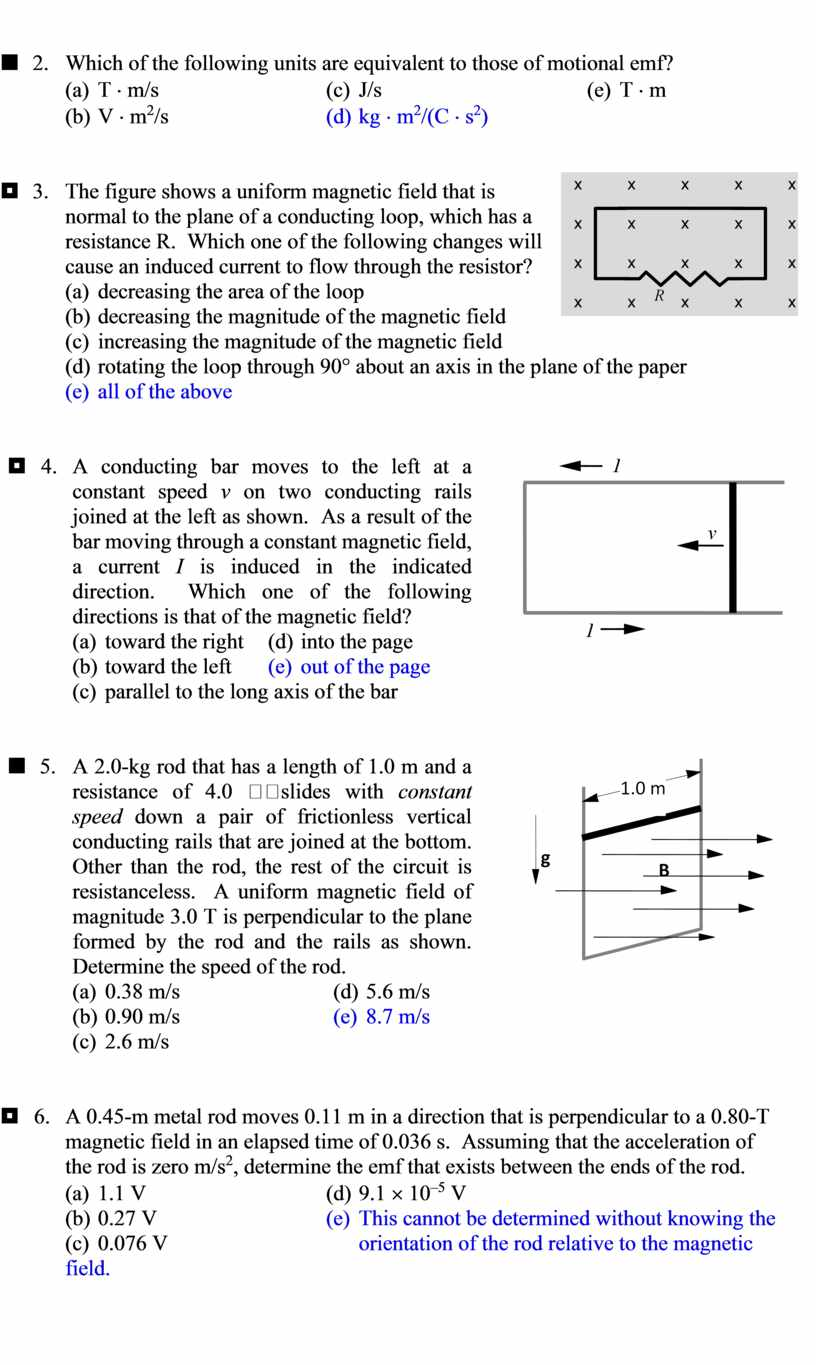## Key 3. The direction of an induced current determines using the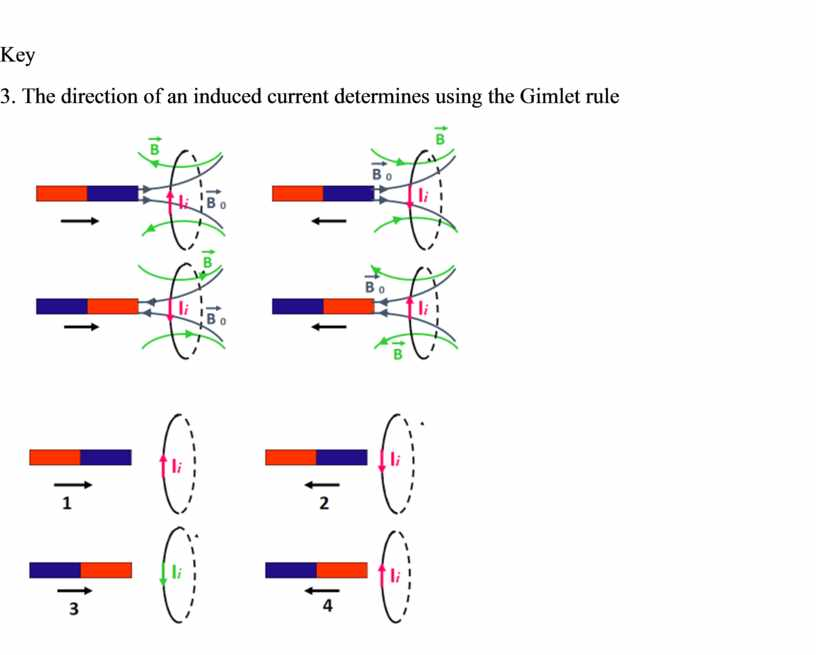Скачать файл